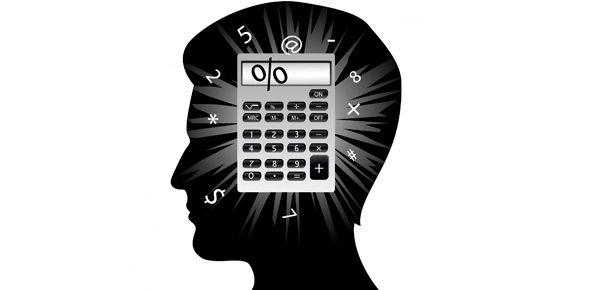# Mental Maths Quiz For Classroom Assistants Assessment for Maths

8 Questions | Total Attempts: 425SettingsClassroom assistants assessment for maths

• 1.
What is 9:30 pm using the 24 hour clock?
• A.

21:30

• B.

20:15

• C.

22:00

• 2.
How amny weeks are in a year?
• A.

12

• B.

52

• C.

36

• 3.
What is a 1/4 as a percentage ?
• A.

60%

• B.

50%

• C.

25%

• 4.
What is 50% as a fraction?
• A.

1/2

• B.

3/4

• C.

2/3/

• 5.
A hexagon has how many sides?
• A.

5

• B.

6

• C.

8

• 6.
How many degrees are in a right angle?
• A.

80

• B.

60

• C.

90

• 7.
What is 3 sguared?
• A.

9

• B.

12

• C.

15

• 8.
What is 20 digrees Celsius in Fahrenheit?
• A.

60

• B.

68

• C.

40

Related Topics